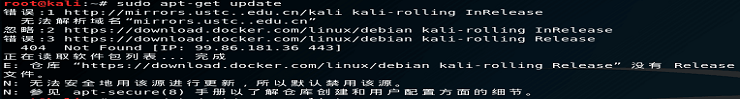# BP: Networking1. How many categories of IPv4 addresses are there?
ANS: 5
2. Which type is for research? *Looking for a letter rather than a number here
Reference: https://www.computerhope.com/jargon/i/ip.htm

ANS: E

3. How many private address ranges are there?
ANS: 3

4. Which private range is typically used by businesses?
ANS: A

5. There are two common default private ranges for home routers, what is the first one?
ANS: 192.168.0.0

6. How about the second common private home range?
ANS: 192.168.1.0

7. How many addresses make up a typical class C range? Specifically a /24
“/24” mean
1111 1111 . 1111 1111 . 1111 1111 . 0000 0000
There’re twenty-four “1”.
There’re eight “0”. → 2⁸ = 256
ANS: 256

8. Of these addresses two are reserved, what is the first addresses typically reserved as?
ANS: Network

9. The very last address in a range is typically reserved as what address type?

10. A third predominant address type is typically reserved for the router, what is the name of this address type?
ANS: Gateway

11. Which address is reserved for testing on individual computers?
ANS: 127.0.0.1

12. A particularly unique address is reserved for unroutable packets, what is that address? This can also refer to all IPv4 addresses on the local machine.
ANS: 0.0.0.0

Calculation method

For an example:
1001 0010 (in binary) = 1*2⁷ + 0*2⁶ + 0*2⁵ + 1*2⁴ + 0*2³ + 0*2² + 1*2¹ + 0*2⁰ = 146 (in decimal)

Calculation method
For an example:
238 (in decimal)
238 / 2 = 119 *2 + 0

Repeat dividing step until you can’t

Fraction in the circle is the answer